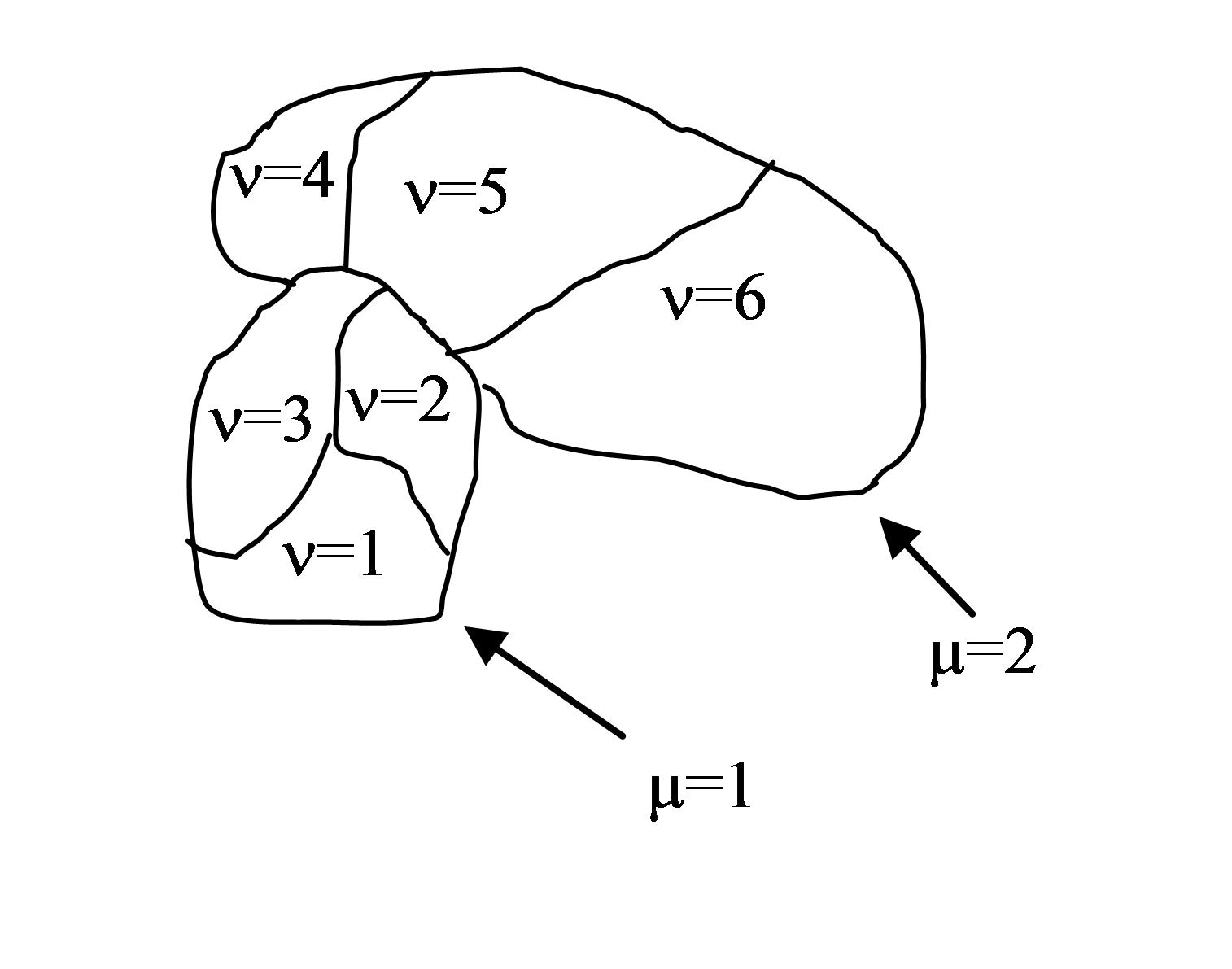# Compartmentalized cells. ContactInternal Plugin¶

Calculating contact energies between compartmentalized cells is analogous to the non-compartmentalized case. The energy expression takes the following form:

\begin{eqnarray} E_{adhesion} = \sum_{i,j,neighbors} J\left ( \sigma (\mu_{i},\nu_{i}),\sigma (\mu_{j},\nu_{j}) \right ) \end{eqnarray}

where i and j denote pixels , σ(µ,ν) denotes a cell type of a cell with cluster id µ and cell id ν . In compartmental cell models a cell is a collection of subcells. Each subcell has a unique id ν (cell id). In addition to that each subcell will have additional attribute, a cluster id (µ) that determines to which cluster of subcells a given subcell belongs. Tthink of a cluster as a cell with nonhomogenous cytoskeleton. So a cluster corresponds to a biological cell and subcells (or compartments) represents parts of a cell e.g. a nucleus. The core idea here is to have different contact energies between subcells belonging to the same cluster and different energies for cells belonging to different clusters. Technically subcells of a cluster are “regular” CompuCell3D cells. By giving them an extra attribute cluster id ((µ) we can introduce a concept of compartmental cells. In our convention σ(0,0) denotes mediumFigure 2. Two compartmentalized cells (cluster id $$\mu=1$$ and cluster id $$\mu=2$$). Compartmentalized cell $$\mu=1$$ consists of subcells with cell id $$\nu=1,2,3$$ and compartmentalized cell $$\mu=2$$ consists of subcells with cell id $$\nu=4,5,6$$

Introduction of cluster id and cell id are essential for the definition of $$J\left ( \sigma (\mu_{i},\nu_{i}),\sigma (\mu_{j},\nu_{j}) \right )$$

Indeed:

\begin{cases} J\left ( \sigma (\mu_{i},\nu_{i}),\sigma (\mu_{j},\nu_{j}) \right ) = J^{external}\left ( \sigma (\mu_{i},\nu_{i}),\sigma (\mu_{j},\nu_{j}) \right ) & \text{ if } \mu_i \neq \mu_j \\ J\left ( \sigma (\mu_{i},\nu_{i}),\sigma (\mu_{j},\nu_{j}) \right ) = J^{internal}\left ( \sigma (\mu_{i},\nu_{i}),\sigma (\mu_{j},\nu_{j}) \right ) & \text{ if } \mu_i = \mu_j \end{cases}

As we can see from above there are two hierarchies of contact energies – external and internal. To describe adhesive interactions between different compartmentalized cells we use two plugins: Contact and ContactInternal. Contact plugin calculates energy between two cells belonging to different clusters and ContactInternal calculates energies between cells belonging to the same cluster. An example syntax is shown below

<Plugin Name="Contact">

<Energy Type1="Base" Type2="Base">0</Energy>
<Energy Type1="Top" Type2="Base">25</Energy>
<Energy Type1="Center" Type2="Base">30</Energy>
<Energy Type1="Bottom" Type2="Base">-2</Energy>
<Energy Type1="Side1" Type2="Base">25</Energy>
<Energy Type1="Side2" Type2="Base">25</Energy>
<Energy Type1="Medium" Type2="Base">0</Energy>

<Energy Type1="Medium" Type2="Medium">0</Energy>
<Energy Type1="Top" Type2="Medium">30</Energy>
<Energy Type1="Bottom" Type2="Medium">20</Energy>
<Energy Type1="Side1" Type2="Medium">30</Energy>
<Energy Type1="Side2" Type2="Medium">30</Energy>
<Energy Type1="Center" Type2="Medium">45</Energy>

<Energy Type1="Top" Type2="Top">2</Energy>
<Energy Type1="Top" Type2="Bottom">100</Energy>
<Energy Type1="Top" Type2="Side1">25</Energy>
<Energy Type1="Top" Type2="Side2">25</Energy>
<Energy Type1="Top" Type2="Center">35</Energy>

<Energy Type1="Bottom" Type2="Bottom">10</Energy>
<Energy Type1="Bottom" Type2="Side1">25</Energy>
<Energy Type1="Bottom" Type2="Side2">25</Energy>
<Energy Type1="Bottom" Type2="Center">35</Energy>

<Energy Type1="Side1" Type2="Side1">25</Energy>
<Energy Type1="Side1" Type2="Center">25</Energy>
<Energy Type1="Side2" Type2="Side2">25</Energy>
<Energy Type1="Side2" Type2="Center">25</Energy>
<Energy Type1="Side1" Type2="Side2">15</Energy>

<Energy Type1="Center" Type2="Center">20</Energy>

<NeighborOrder>2</NeighborOrder>
</Plugin>


and

<Plugin Name="ContactInternal">

<Energy Type1="Base" Type2="Base">0</Energy>
<Energy Type1="Base" Type2="Bottom">0</Energy>
<Energy Type1="Base" Type2="Side1">0</Energy>
<Energy Type1="Base" Type2="Side2">0</Energy>
<Energy Type1="Base" Type2="Center">0</Energy>

<Energy Type1="Top" Type2="Top">4</Energy>
<Energy Type1="Top" Type2="Bottom">25</Energy>
<Energy Type1="Top" Type2="Side1">22</Energy>
<Energy Type1="Top" Type2="Side2">22</Energy>
<Energy Type1="Top" Type2="Center">15</Energy>

<Energy Type1="Bottom" Type2="Bottom">4</Energy>
<Energy Type1="Bottom" Type2="Side1">15</Energy>
<Energy Type1="Bottom" Type2="Side2">15</Energy>
<Energy Type1="Bottom" Type2="Center">10</Energy>

<Energy Type1="Side1" Type2="Side1">11</Energy>
<Energy Type1="Side2" Type2="Side2">11</Energy>
<Energy Type1="Side1" Type2="Side2">11</Energy>

<Energy Type1="Side2" Type2="Center">10</Energy>
<Energy Type1="Side1" Type2="Center">10</Energy>

<Energy Type1="Center" Type2="Center">2</Energy>

<NeighborOrder>2</NeighborOrder>
</Plugin>


Depending whether pixels for which we calculate contact energies belong to the same cluster or not we will use internal or external contact energies respectively.